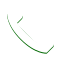# How to Measure

How much sod do I need? Knowing how much sod you need is pivotal to the ordering process. We are a sod service in Orlando who is more than happy to educate you in the measurement process. To find out the quantity of sod you need, you must first measure the area(s) that you are going to sod. Start by turning the area(s) that you are sodding into shapes that can be measured without much difficulty. Here are some basic shapes and how to measure each of them. Once you have your total square footage, give our professional and courteous staff a call and they will help you with the rest.

### SQUARE or RECTANGULARMeasure the length and width in feet. Multiply these two numbers together. The resulting number is the total square footage that is required. If you have more than one area, repeat this process and ADD each total area together.

### TRIANGLEMeasure the longest base and the height from the base of the shape. Divide the result by 2. The resulting number is the total square footage that is required. If you have more than one area, repeat this process and ADD each total area together.

### CIRCLEMeasure the diameter of your circle. Divide this number by 2. The result is the radius (r). Multiply the radius by the radius (not x2), then multiply it by 3.14. The resulting number is the total square footage that is required. If you have more than one area, repeat this process and ADD each total area together.

### TRAPEZOIDMeasure the bottom length of your trapezoid (b). Measure the top length of your trapezoid (a). Add these two amounts together (a + b) then divide this number by 2. Multiply this result by h, which is the height of your trapezoid. The resulting number is the total square footage that is required. If you have more than one area, repeat this process and ADD each Total area together.

### PARALLELOGRAMMeasure the bottom length of your Parallelogram (b). Multiply this result by h, which is the height of your parallelogram. The resulting number is the total square footage that is required. If you have more than one area, repeat this process and ADD each Total area together.Call Today